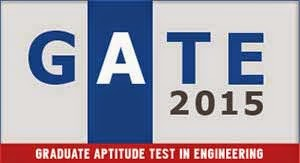# GATE 2015 syllabus, GATE 2015 syllabus for ece, Gate Syllabus for Electronics Engineering, Gate syllabus for Electronics and Communication Engineering, syllabus for electronics and communication engineering, gate syllabus for etc, Gate syllabus for Electronics and TeleCommunication# GATE 2015 syllabus for ECE

### Syllabus for Electronics and Communication Engineering (ECE)

Engineering Mathematics

Linear Algebra:
Matrix Algebra, Systems of linear equations, Eigen values and eigen vectors.

Calculus:
Mean value theorems, Theorems of integral calculus, Evaluation of definite and improper
integrals, Partial Derivatives, Maxima and minima, Multiple integrals, Fourier series. Vector
identities, Directional derivatives, Line, Surface and Volume integrals, Stokes, Gauss and

Green's theorems.

Differential equations:
First order equation (linear and nonlinear), Higher order linear differential equations with
constant coefficients, Method of variation of parameters, Cauchy's and Euler's equations, Initial
and boundary value problems, Partial Differential Equations and variable separable method.

Complex variables:
Analytic functions, Cauchy's integral theorem and integral formula, Taylor's and Laurent' series,
Residue theorem, solution integrals.

Probability and Statistics:
Sampling theorems, Conditional probability, Mean, median, mode and standard deviation,
Random variables, Discrete and continuous distributions, Poisson, Normal and Binomial
distribution, Correlation and regression analysis.

Numerical Methods:
Solutions of non-linear algebraic equations, single and multi-step methods for differential
equations.

Transform Theory:
Fourier transform, Laplace transform, Z-transform.

Electronics and Communication Engineering
Networks:
Network graphs: matrices associated with graphs; incidence, fundamental cut set and
fundamental circuit matrices. Solution methods: nodal and mesh analysis. Network theorems:
superposition, Thevenin and Norton's maximum power transfer, Wye-Delta transformation.
Steady state sinusoidal analysis using phasors. Linear constant coefficient differential equations;
time domain analysis of simple RLC circuits, Solution of network equations using Laplace
transform: frequency domain analysis of RLC circuits. 2-port network parameters: driving point
and transfer functions. State equations for networks.

Electronic Devices:
Energy bands in silicon, intrinsic and extrinsic silicon. Carrier transport in silicon: diffusion
current, drift current, mobility, and resistivity. Generation and recombination of carriers. p-n
junction diode, Zener diode, tunnel diode, BJT, JFET, MOS capacitor, MOSFET, LED, p-I-n
and avalanche photo diode, Basics of LASERs. Device technology: integrated circuits
fabrication process, oxidation, diffusion, ion implantation, photolithography, n-tub, p-tub and
twin-tub CMOS process.

Analog Circuits:
Small Signal Equivalent circuits of diodes, BJTs, MOSFETs and analog CMOS. Simple diode
circuits, clipping, clamping, rectifier. Biasing and bias stability of transistor and FET amplifiers.
Amplifiers: single-and multi-stage, differential and operational, feedback, and power. Frequency
response of amplifiers. Simple op-amp circuits. Filters. Sinusoidal oscillators; criterion for
oscillation; single-transistor and op-amp configurations. Function generators and wave-shaping
circuits, 555 Timers. Power supplies.

Signals and Systems:
Definitions and properties of Laplace transform, continuous-time and discrete-time Fourier
series, continuous-time and discrete-time Fourier Transform, DFT and FFT, z-transform.
Sampling theorem. Linear Time-Invariant (LTI) Systems: definitions and properties; causality,
stability, impulse response, convolution, poles and zeros, parallel and cascade structure,
frequency response, group delay, phase delay. Signal transmission through LTI systems.

Communications:
Random signals and noise: probability, random variables, probability density function,
autocorrelation, power spectral density. Analog communication systems: amplitude and angle
modulation and demodulation systems, spectral analysis of these operations, superheterodyne
receivers; elements of hardware, realizations of analog communication systems; signal-to-noise
ratio (SNR) calculations for amplitude modulation (AM) and frequency modulation (FM) for low
noise conditions. Fundamentals of information theory and channel capacity theorem. Digital
communication systems: pulse code modulation (PCM), differential pulse code modulation
(DPCM), digital modulation schemes: amplitude, phase and frequency shift keying schemes
(ASK, PSK, FSK), matched filter receivers, bandwidth consideration and probability of error
calculations for these schemes. Basics of TDMA, FDMA and CDMA and GSM.

Electromagnetics:
Elements of vector calculus: divergence and curl; Gauss' and Stokes' theorems, Maxwell's
equations: differential and integral forms. Wave equation, Poynting vector. Plane waves:
propagation through various media; reflection and refraction; phase and group velocity; skin
depth. Transmission lines: characteristic impedance; impedance transformation; Smith chart;
impedance matching; S parameters, pulse excitation. Waveguides: modes in rectangular
waveguides; boundary conditions; cut-off frequencies; dispersion relations. Basics of
propagation in dielectric waveguide and optical fibers. Basics of Antennas: Dipole antennas;